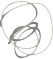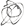Noppanit

02 Jan 2018

# So, You Want to Understand Recursion - Part one

I’m studying recursion as part of my learning about Algorithms and Data Structures. This is my attempt to explain the steps I took to learn the topic and I hope it will benefit anyone who comes across this post in the future.

For those who are unfamiliar with recursion, here is a good introductory video that really captures the history and core concepts of recursion.

I have also found this site that helps in visualizing the step-by-step process of how a recursive program works. Alternatively, you can use a debugger on your IDE.

This is an example of the visualization.

Of all the books and websites I’ve come across, the most efficient method to learn about recursion is to visualize the execution of the program and practice A LOT.

Recursion is an important foundational concept for other complex algorithms such as Dynamic Programming, Backtracking or Divide and Conquer, which I’ll cover in the upcoming posts. It will benefit you greatly if you understand the fundamentals of this simple algorithm. So, let’s dive in.

### Types of Recursions

• Linear recursion

Linear recursion is a function where a method calls itself only once. An example of this type of recursion is the factorial problem.

• Tail recursion

Tail recursion is the same as Linear recursion, but calling the recursion is the last operation of the function. For example:


def print(n):
if n == 0:
return
print(n)
print(n - 1)


• Multiple recursion

Multiple recursion happens when a method calls itself multiple times. An example of this type of recursion is the fibonacci number.

• Mutual recursion

Mutual recursion happens when functions call each other.

• Nested recursion

Ackermann function is an example of nested recursion where the argument of the function is the function itself. I won’t go into the details of this function since I haven’t found many cases of this type of recursion except for this

It is not necessary to memorize the different types of recursion as it won’t really help you to solve the problem, however, it is good to know the keywords so that you can use them to do a web-search on the problem later on.

### The factorial problem.

Factorial is the product of all positive integers less than or equal to n. For example:


5! = 5 x 4 x 3 x 2 x 1



It could be written in Python like this:


def factorial(n):
if n == 0:
return 1
else:
return n * factorial(n-1)



The code reads like this: When n equals to 0 we return 1, otherwise, we return a product of n and factorial(n-1), which means we subtract n by 1 and call the function again. We repeat this process until the number equals to 0, when the function will start to unwind.

### How to solve recursion.

#### Base case

What does base case mean? Every recursion function needs to have at least one base case to end the program. Otherwise, the program will run forever and eventually it will be terminated by your compiler. For example, if you run the following in your IDE:


def factorial(n):
return n * factorial(n-1)



You will get error like this:


RecursionError: maximum recursion depth exceeded



And almost all recursion functions will have a skeleton/template like this:


def function(some_arguments):
if (base case):
// do something
else
// call itself or other function



#### Sub problem

What does it mean? It means that your problem has to be able to be broken down into smaller problems in order for the problem to be solved recursively.

For example, in the factorial function, the smaller version of factorial(5) is factorial(5-1). If you want to write a program to sum the values from an array of integers such as:


nums = [1, 2, 3, 4, 5]



, the sub problem of this program would be that we need to make the array smaller by increasing the position/index of the array. When the index hits the end of the array and as the program unwinds we then add up the number being returned from the function.


def summation(nums, size):
if size == 0:
return nums[size]
return summation(nums, size - 1) + nums[size]

if __name__ == '__main__':
nums = [1, 2, 3, 4, 5, 6]
print(summation(nums, len(nums)))



In conclusion, when you approach a recursion problem, you need to be thinking: how can I break this problem down into smaller chunks, and as the program unwinds how can I capture the result of each small problem?

### Reference

#### Books

Til next time,
noppanit at 21:29